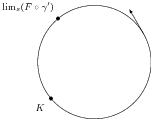phystricks
phystricks : use python to create your pictures to be inserted in LaTeX

## General introduction

The purpose of this module is to produce pictures to be inserted in your LaTeX document using only python and Sage techniques. The motto is :

«*if Sage can compute it, LaTeX can draw it*»

### What is LaTeX ? What is tikz ?

If you don't know what is LaTeX, simply read the description on wikipedia. For the moment you just have to know that LaTeX is a text editor with the same ideas of markdown or wiki, except that

• the mainstream output of LaTeX is pdf (which is static) while markdown or wiki will output an html file which is more dynamic.
• LaTeX is provided with a "programming language" of macros. One can do some "if-then-else".

tikz is a set of macros for creating pictures in LaTeX. The weakness of Tikz for producing scientific pictures are :

• no (reasonable) ways to create loops
• no math : cannot compute more than some classical functions, no way to compute the derivative, numerical integrals, ...
• no (reasonable) ways to declare a variable and use it in the definition of a function or in the computation of the coordinates of a point

### What do people do ?

People use an external program to produce the pictures and then include them as pdf, png or worse : ps ... even worse : epsi.

What's the problem with this approach ? If you want to name a point "A", it will appears with the font of the external program, not the font of you current LaTeX file, and you cannot use the LaTeX macros in the picture.

## What is phystricks

### What problem do we solve ?

Including complex figures in LaTeX is always difficult because you

• want use an external program because LaTeX as "programming language" is by far too complicated,
• want add labels that contains mathematical formulas; and these labels should be compiled by LaTeX, not by your external program,
• for some reasons, don't (want to) know ps stuff like psfrag.

### How do we solve ?

phystricks is a python (Sage in fact) module defining classes like point, segment, parametric curve, ... and many geometric relations between them. You describe your picture using Python and phystricks creates the tikz code to be included in your LaTeX file.

### Example

1 # -*- coding: utf8 -*-
2
3 from phystricks import *
4 def VSJOooJXAwbVEt():
5  pspict,fig = SinglePicture("VSJOooJXAwbVEt")
6  pspict.dilatation(1)
7
8  O=Point(0,0)
9
10  circle=Circle( O,2 ) # center, radius
11
12  # Points are parametrized by their angle (degree)
13  A=circle.get_point(130)
14  B=circle.get_point(220)
15  tg=circle.get_tangent_vector(30)
16
17  # dist : the distance between the circle and the mark.
18  # text : the LaTeX code that will be placed there.
19  A.put_mark(dist=0.3,text="$\lim_{s}(F\circ\gamma')$",pspict=pspict)
20  B.put_mark(dist=0.3,text="$K$",pspict=pspict)
21
22  pspict.DrawGraphs(circle,A,tg,B)
23
24  fig.no_figure()
25  fig.conclude()
26  fig.write_the_file()

The you compile the picture with Sage :

1 ┌────────────────────────────────────────────────────────────────────┐
2 │ SageMath Version 7.0, Release Date: 2016-01-19 │
3 │ Type "notebook()" for the browser-based notebook interface. │
4 │ Type "help()" for help. │
5 └────────────────────────────────────────────────────────────────────┘
6 sage: attach("<filename>.py")
7 sage: VSJOooJXAwbVEt()

Now the file Fig_VSJOooJXAwbVEt.pstricks is created and you just have to add the following lines in you LaTeX document :

1 \begin{center}
2  \input{Fig_VSJOooJXAwbVEt.pstricks}
3 \end{center}

What you get is :As you see, taking the tangent vector is a simple as calling the method get_tangent_vector with as argument the angle on the circle.

Notice that :

• The labels are well placed : they are in such a way that they will not intersect the circle.
• At no point the Sage code speaks about the size of the box containing the labels.

The trick is that phystricks does not only produces the tikz code for the picture, but also make LaTeX write the size of the box in an auxiliary file. Thus in a second pass of phystricks, the size of the box is known and the label can be correctly placed.

The LaTeX code inserted in your picture is compiled by LaTeX in the same time as your document.